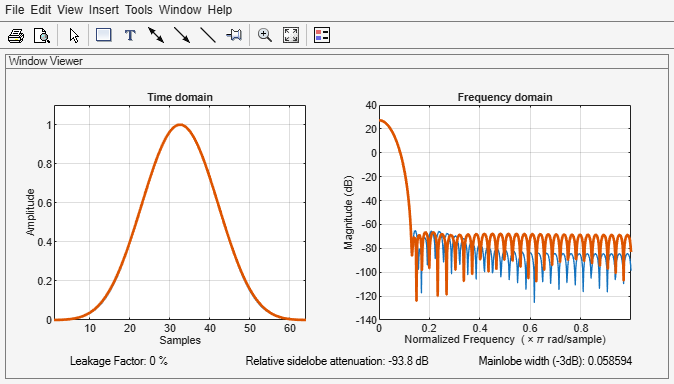# nuttallwin

Nuttall-defined minimum 4-term Blackman-Harris window

## Syntax

```w = nuttallwin(N) w = nuttallwin(N,SFLAG) ```

## Description

`w = nuttallwin(N)` returns a Nuttall defined `N`-point, 4-term symmetric Blackman-Harris window in the column vector `w`. The window is minimum in the sense that its maximum sidelobes are minimized. The coefficients for this window differ from the Blackman-Harris window coefficients computed with `blackmanharris` and produce slightly lower sidelobes.

`w = nuttallwin(N,SFLAG)` uses `SFLAG` window sampling. `SFLAG` can be `'symmetric'` or `'periodic'`. The default is `'symmetric'`. You can find the equations defining the symmetric and periodic windows in Algorithms.

## Examples

collapse all

Compare 64-point Nuttall and Blackman-Harris windows. Plot them using `wvtool`.

```L = 64; w = blackmanharris(L); y = nuttallwin(L); wvtool(w,y)```Compute the maximum difference between the two windows.

`max(abs(y-w))`
```ans = 0.0099 ```

## Algorithms

The equation for the symmetric Nuttall defined four-term Blackman-Harris window is

`$w\left(n\right)={a}_{0}-{a}_{1}\mathrm{cos}\left(2\pi \frac{n}{N-1}\right)+{a}_{2}\mathrm{cos}\left(4\pi \frac{n}{N-1}\right)-{a}_{3}\mathrm{cos}\left(6\pi \frac{n}{N-1}\right)$`

where n= 0,1,2, ... N-1.

The equation for the periodic Nuttall defined four-term Blackman-Harris window is

`$w\left(n\right)={a}_{0}-{a}_{1}\mathrm{cos}\left(2\pi \frac{n}{N}\right)+{a}_{2}\mathrm{cos}\left(4\pi \frac{n}{N}\right)-{a}_{3}\mathrm{cos}\left(6\pi \frac{n}{N}\right)$`

where n= 0,1,2, ... N-1. The periodic window is N-periodic.

The coefficients for this window are

a0 = 0.3635819

a1 = 0.4891775

a2 = 0.1365995

a3 = 0.0106411

## References

 Nuttall, Albert H. “Some Windows with Very Good Sidelobe Behavior.” IEEE® Transactions on Acoustics, Speech, and Signal Processing. Vol. ASSP-29, February 1981, pp. 84–91.